# Artificial Neural Networks: The Foundation of ML

This article introduces the feed-forward neural network, its underlying structure and how a “forward pass” (generating an output from input data) is performed within it. This article acts as an introduction to neural networks for those who have not endeavoured into them before, and provides a foundational understanding by which all other neural networks are built upon.

A feed-forward neural network is the most basic type of Artificial Neural Network (ANN). They consist of an input layer, 1 or more hidden layers, and an output layer. Each layer consists of neurons, and neurons also have their own structure. We will not only be covering the components of a neuron, but also how neurons are connected throughout a network. It is this formation that essentially derives the term neural network.

Once the network structure is covered, we will test-drive it. That is to say, we will be providing input data into the neural network, and analyse exactly what is happening throughout the layers until an output value is generated.

Note that we will assume the networks used here are already trained (the process of optimising the network to efficiently achieve its task). That is to say, our network will already be configured to perform its task well, and that task will be to compute a logical AND operation. This operation will be explained further down when we delve into the calculations of a complete forward pass.

Concretely, we will be endeavouring into neural networks by:

• Explaining the structure of a neural network and its layers.
• Breaking apart the components of a neuron. Each neuron in a neural network manipulates data to various degrees. To explain this process, concepts such as weight, activation functions and bias will be introduced to the reader.
• Calculating an entire forward pass through a relatively simple neural network, trained to carry out a logical AND operation.

Let’s start with the high-level structure of a neural network, and then break down the components of a neuron.

# The Neural Network

A neural network is simply a network of “neurons” that are connected at various points throughout the network. The neural network we’ll be looking at here is one consisting of fully connected layers, that is to say, every neuron in one layer is connected to every neuron in the previous layer and next layer.

A neural network can be thought of as a function. But instead of explicitly defining the function like we would in a traditional programming paradigm, we “train” or “optimise” the network to mimic a function as accurately as possible. Each neuron handles some logic about the function, before the output neuron generates a final return value. This makes neural networks predictive, rather than absolute, in nature. This will become apparent further down.

The general structure of a neural network can be summarised as follows: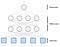Structure of a feed-forward neural network.

Consider the above diagram. Imagine your eyes giving your input nodes data, which are then processed through your brain’s neurons, before a final cognitive thought is concluded thereafter, with this step representing the output layer.

The human brain has ~86 billion neurons (>100 billion with some estimates), each of which are connected to ~1000 others. With the current speed at which consumer-oriented ML chips are evolving every year, it will be around 2024 when ML chips will support ANNs that match or surpass this estimated human brain capacity. Even though artificial neurons indeed work differently to biological neurons, this milestone will nonetheless be huge for AI.

As we can clearly see, a neural network consists of 3 types of layers:

• Input layer: Provides 1 or more nodes in the network to accept input values. These nodes are not neurons — they represent the data we are feeding into the network. This is why they are squares and not circles.
• Hidden layer(s): Hidden layers consist of neurons that exist between the input layer and output layer. This is where the bulk of calculations take place before any output value is generated.
• Output layer: In feed-forward neural networks, the output layer is usually one neuron, that determines the final output generated by the network. The output layer can output a number of results, such as a binary 1 or 0, a classification and even a probability distribution.

As an intuitive classification example of how a neural network operates, imagine feeding the network a large amount of characteristics of a particular animal. The network will feed this input data throughout the network, and is manipulated along the way (by means we will discuss next) before an output neuron will generate the classification — in this example, of which species of the animal in question.

With this in mind, let’s now delve deeper into what actually comprises a neuron.

# Linear and Nonlinear Perceptrons

A neuron in feed-forward neural networks come in two forms — they either exist as linear perceptrons or nonlinear perceptrons. Just about all neural networks you will encounter will have neurons in the form of nonlinear perceptrons, because as the name suggests, the output of the neuron becomes nonlinear. This section will delve into what this all means and the differences between the two. In fact, and as we will see, a nonlinear perceptron is just a linear perceptron with an additional component attached to it to further process its output.

## The Linear Perceptron

A linear perceptron can either output a value of `0` or `1` — let’s begin to explore how this is achieved. The following illustration shows the components of a linear perceptron: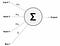Structure of the linear perceptron.

Inputs 1–3 represent the values from the previous neuron outputs (which in this case represents the previous layer). We have also introduced some weights (w) for each input, that scale each input by varying degrees. After we’ve “scaled” each input, they get summed up at the neuron.

Weights are learned values, that is to say, they are derived when performing a training algorithm on the network, such as back propagation. In this article we are assuming our network is fully trained already, with the weight values reflecting their optimal values for the network.

Notice that we’ve also introduced a bias value (b) that is fed into the neuron to further scale the summed value at the neuron. The bias value is always `1`, and is also called the activation threshold. This is because the summed neuron value must be greater than this threshold to produce a `1` output. We can summarise this rule as follows: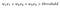The summed neuron value must be greater than threshold to produce an output.

This equation can be re-written by moving the threshold to the left side.This effectively treats the bias value as just another weight: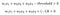Re-writing the linear perceptron equation, treating bias as another weight.

So our scaled inputs and bias are fed into the neuron and summed up, which then result in a `0` or `1` output value — in this case, any value above `0` will produce a `1`.

Binary neurons (0s or 1s) are interesting, but limiting in practical applications. Let’s now expand our understanding of the neuron by adding a nonlinear component to it, that changes this binary output to a ranged output.

## The Nonlinear Perceptron

Now, there are some limitations of linear perceptrons, and these limitations are well understood.

Linear perceptrons allow continuous functions to be calculated via a neural network (think of a sin curve, or similar continuous functions that have that linear attribute), but linear perceptrons can not account for all functions, such as classification tasks, one of the most common use cases of neural networks. In other words, they are not universal function approximators — they cannot represent some functions.

Another limitation of linear perceptrons is that they are not differentiable, and this becomes an issue when training the network, as there is no means of gradually minimising error, or loss, until an optimal is found. Manually trying to find optimal weights for a neural network would not be fun, and would not take any realistic amount of time with larger networks — we therefore want to be able to train the network with a suitable algorithm!

If a neuron instead outputted a differentiable signal that could be gradually tweaked to improve the performance of the network, it could effectively be trained to an optimal value. What we need is an additional component to the linear perceptron to support a range of values between 0 and 1 — and that is what the nonlinear activation function is for.

Nonlinear activation functions are calculated by scaling the weights of each input and adding its bias value like before. What we do with these values now is pass them into another function to generate values between 0 and 1.

A commonly used nonlinear activation function at the introductory level is the sigmoid function.

The sigmoid function takes an input value and outputs a value between 0 and 1. It is a rather simple and cheap activation function to use, and comes in the form of the following equation, where z is the neuron’s scaled summed output: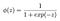The sigmoid function, with z as its only parameter.

z here represents the result of the linear perceptron calculation. One can plug any float value into z and affirm that the resulting value will always be between 0 and 1.

With that being said, let’s update our neuron to reflect this additional nonlinear component: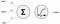A nonlinear perceptron with a sigmoid activation function

The sigmoid function is by no means the only nonlinear activation function, and there are others (such as ReLU and TanH), that come with their own curve behaviour and perform better for certain use cases.

The final neuron output is also referred to as the neuron’s activation, annotated a.

The nonlinear perceptron calculation can be summarised by either considering a singular neuron, or more commonly an entire layer of neurons. These formulas are as follows, with the latter in matrix form: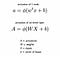Activation Vector = Sigmoid((weights * inputs) + bias))

This simply means we are passing the result of the scaled inputs plus the bias value into our nonlinear activation function ϕ, which in this piece has been the sigmoid function. The latter formula is preferred over the former, whereby A will contain a vector of all the sigmoid activations of the layer, each corresponding to a neuron in that layer.

If you have not worked with matrices before and would like to brush up on fundamental matrix operations, I have published an article to introduce working with matrices to the reader. Find that article here: AI Essentials: Working with Matrices.

We have now covered the neuron for a feed-forward neural network, with the output of our nonlinear activation function being the “official” output of the neuron. In the final section of this piece, we’ll see how a network of nonlinear perceptrons calculate an output with a forward pass through a neural network.

# Neural Network Example

Now we will be using a small trained neural network to represent a logical AND operation. A logical AND operation takes 2 inputs, and only returns `1` when both inputs are `1`, otherwise it returns `0`. The following table represents all outputs corresponding to all possible inputs: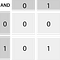Logical AND table

The neural network to process this operation will have 2 input nodes, a hidden layer of 3 neurons, and an output layer of 1 neuron. Each edge is accompanied with weights, and each neuron with bias values: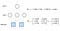The Logical AND neural network with 2 layers, their weights and bias values.

These weights are trained values that are optimal for this particular neural network.

Matrix notation has been introduced here to demonstrate how to bundle all the weights and bias values for a particular layer. This is common practice when programatically designing neural networks.

The `W1` matrix has a shape of 3 x 2, whereby each input has a corresponding weight value for each neuron in layer 1. The `b1` matrix contains 3 elements that correspond to the bias values of each neuron of layer 1.

The 3 neurons of layer 1 will generate 3 output values that are fed into the final layer, with `W2` representing the weights of those 3 values. Finally, `b2` is the bias value of the output neuron.

Let’s now go ahead and calculate a full forward pass, remembering that each neuron is a nonlinear perceptron, which performs the process of summing the scaled values, followed by the sigmoid function.

# Calculating a Forward Pass

To make the following calculations easier to follow, the weight and bias values will be rounded to 1 decimal place.

Again, to brush up on matrix multiplication and addition for the section to follow, check out my introductory article here: AI Essentials: Working with Matrices.

## Layer 1 calculations

The 2 inputs of the neural network will be `1` and `1`. By referencing the above logical AND table, we should expect the network to generate something around `1` as its output.

The inputs of layer 1 are firstly processed, whereby they are scaled by the corresponding weights and subject to the biases:

Element-wise sigmoid is then carried out on this resulting Z vector, providing the final outputs of layer 1:

The resulting vector holds the “activations” of layer 1, and are now passed to the single neuron of layer 2. This is reflected in the illustration above, whereby the 3 neurons of layer 1 are connected to layer 2.

## Layer 2 calculations

Let’s finally calculate the output of layer 2 to retrieve the final output of the network:

And again, we pass `z` (now a single value) into a sigmoid function to yield the final result:

Notice that the final result of the network is not 1, but 0.999… This reflects the predictive nature of neural networks; they only ever produce estimated values based on how it was trained. Understanding this nature is key in understanding and leveraging neural networks effectively.

But saying that, the network does indeed output the correct result when performing a logical AND operation with `1` and `1` as inputs!

# In Summary

This article has aimed to introduce the reader to neural networks, what they are exactly, and to explain the fundamental feed-forward neural network.

The linear and nonlinear perceptron were explained, with the latter solving the limitations of the former by introducing a nonlinear activation function. The benefits to doing this will be evident as you begin training networks, but ultimately, activation functions allow a range of values instead of a binary 0 or 1 output that the linear perceptron yields.

We then put this knowledge to the test by performing a full “forward pass”: the process of passing inputs through a neural network and generating a resulting value in the output layer. With the understanding of this article, the reader is more than equipped to explore:

• More nonlinear activation functions, and how they differ from the sigmoid function.
• The training process of neural networks, such as back propagation, that optimise a network’s weights.
• More complex neural networks such as Recurrent Neural Networks (RNN), a natural next step after the feed-forward network is understood.

Programmer and Author. Director @ JKRBInvestments.com. Creator of LearnChineseGrammar.com for iOS.

## More from Ross Bulat

Programmer and Author. Director @ JKRBInvestments.com. Creator of LearnChineseGrammar.com for iOS.